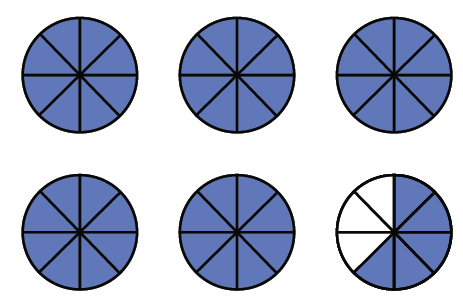### Home > MC1 > Chapter 6 > Lesson 6.2.1 > Problem6-50

6-50.

Answer each of the questions below.

1. How many eighths are in $5\frac{5}{8}$? Use your answer to rewrite $5\frac{5}{8}$ in the form $\frac { \square } { 8 }$.2. How many fifths are in $6\frac{4}{5}$? Use your answer to rewrite $6\frac{4}{5}$ in the form $\frac { \square } { 5 }$.
$34, \frac{34}{5}$